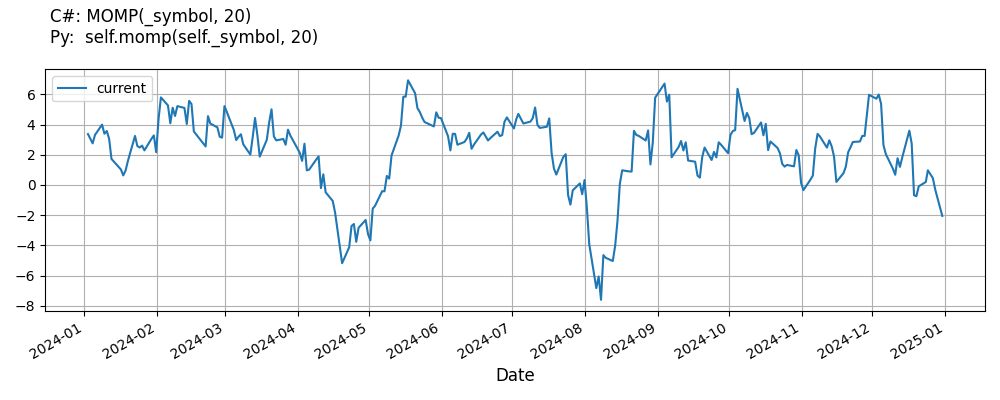# Supported Indicators

## Momentum Percent

### Introduction

This indicator computes the n-period percentage rate of change in a value using the following: 100 * (value_0 - value_n) / value_n This indicator yields the same results of RateOfChangePercent

To view the implementation of this indicator, see the LEAN GitHub repository.

### Using MOMP Indicator

To create an automatic indicators for MomentumPercent, call the MOMP helper method from the QCAlgorithm class. The MOMP method creates a MomentumPercent object, hooks it up for automatic updates, and returns it so you can used it in your algorithm. In most cases, you should call the helper method in the Initialize method.

public class MomentumPercentAlgorithm : QCAlgorithm
{
private Symbol _symbol;
private MomentumPercent _momp;

public override void Initialize()
{
_momp = MOMP(_symbol, 20);
}

public override void OnData(Slice data)
{
{
// The current value of _momp is represented by itself (_momp)
// or _momp.Current.Value
Plot("MomentumPercent", "momp", _momp);

}
}
}
class MomentumPercentAlgorithm(QCAlgorithm):
def Initialize(self) -> None:
self.momp = self.MOMP(self.symbol, 20)

def OnData(self, slice: Slice) -> None:
# The current value of self.momp is represented by self.momp.Current.Value
self.Plot("MomentumPercent", "momp", self.momp.Current.Value)



The following reference table describes the MOMP method:

### MOMP()1/1

            MomentumPercent QuantConnect.Algorithm.QCAlgorithm.MOMP (
Symbol                           symbol,
Int32                            period,
*Nullable<Resolution>      resolution,
*Func<IBaseData, Decimal>  selector
)


Creates a new MomentumPercent indicator. This will compute the n-period percent change in the security. The indicator will be automatically updated on the given resolution.

If you don't provide a resolution, it defaults to the security resolution. If you provide a resolution, it must be greater than or equal to the resolution of the security. For instance, if you subscribe to hourly data for a security, you should update its indicator with data that spans 1 hour or longer.

You can manually create a MomentumPercent indicator, so it doesn't automatically update. Manual indicators let you update their values with any data you choose.

Updating your indicator manually enables you to control when the indicator is updated and what data you use to update it. To manually update the indicator, call the Update method with time/number pair, or an IndicatorDataPoint. The indicator will only be ready after you prime it with enough data.

public class MomentumPercentAlgorithm : QCAlgorithm
{
private Symbol _symbol;
private MomentumPercent _momp;

public override void Initialize()
{
_momp = new MomentumPercent(20);
}

public override void OnData(Slice data)
{
if (data.Bars.TryGeValue(_symbol, out var bar))
{
_momp.Update(bar.EndTime, bar.Close);
}

{
// The current value of _momp is represented by itself (_momp)
// or _momp.Current.Value
Plot("MomentumPercent", "momp", _momp);

}
}
}
class MomentumPercentAlgorithm(QCAlgorithm):
def Initialize(self) -> None:
self.momp = MomentumPercent(20)

def OnData(self, slice: Slice) -> None:
bar = slice.Bars.get(self.symbol)
if bar:
self.momp.Update(bar.EndTime, bar.Close)

# The current value of self.momp is represented by self.momp.Current.Value
self.Plot("MomentumPercent", "momp", self.momp.Current.Value)



To register a manual indicator for automatic updates with the security data, call the RegisterIndicator method.

public class MomentumPercentAlgorithm : QCAlgorithm
{
private Symbol _symbol;
private MomentumPercent _momp;

public override void Initialize()
{
_momp = new MomentumPercent(20);
RegisterIndicator(_symbol, _momp, Resolution.Daily);
}

public override void OnData(Slice data)
{
{
// The current value of _momp is represented by itself (_momp)
// or _momp.Current.Value
Plot("MomentumPercent", "momp", _momp);

}
}
}
class MomentumPercentAlgorithm(QCAlgorithm):
def Initialize(self) -> None:
self.momp = MomentumPercent(20)
self.RegisterIndicator(self.symbol, self.momp, Resolution.Daily)

def OnData(self, slice: Slice) -> None:
# The current value of self.momp is represented by self.momp.Current.Value
self.Plot("MomentumPercent", "momp", self.momp.Current.Value)



The following reference table describes the MomentumPercent constructor:

### MomentumPercent()1/2

            MomentumPercent QuantConnect.Indicators.MomentumPercent (
int  period
)


Creates a new MomentumPercent indicator with the specified period.

### MomentumPercent()2/2

            MomentumPercent QuantConnect.Indicators.MomentumPercent (
string  name,
int     period
)


Creates a new MomentumPercent indicator with the specified period.

### Visualization

The following image shows plot values of selected properties of MomentumPercent using the plotly library.You can also see our Videos. You can also get in touch with us via Discord.